# Discount

The new phone was discounted by 2800kč. After this discount, 5 phones cost 5530 CZK, more than 3 phones before the discount. How much did the phone cost before the discount?

x =  9765

### Step-by-step explanation:

5•(x-2800) = 3•x + 5530

2x = 19530

x = 9765

Our simple equation calculator calculates it.Did you find an error or inaccuracy? Feel free to write us. Thank you!Tips to related online calculators
Do you have a linear equation or system of equations and looking for its solution? Or do you have a quadratic equation?

## Related math problems and questions:

• Discount saleAfter the discount, the computer costs 9600, - CZK. How much did it cost when the price was reduced: a) by half b) by a third c) by one fifth and then by 160 CZK
• Czech coins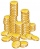John has 540 CZK, but he has 10 CZK coins and 20 CZK coins only, we knows that he has 10CZK coins 7times more that 20CZK coins, how many 10CZK coin and how many 20CZK coins he saved?
• Double ratio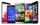The mobile phone was twice gradually discounted in the ratio of 3: 2 1 half: 5 quarters. How much did it originally cost if the price was CZK 4,200 after a double discount?
• Performance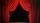Same number of tickets sold for 15 CZK, 20 CZK, 30 CZK and 40 CZK a total of CZK 13,230 on theatre performance. How many tickets were sold for this performance?
• Birthday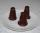Mother bought 21 desserts on the occasion of Mirka's birthday one tips was 9 CZK and the kremlin cost 12 CZK. For all desserts, she paid 213 CZK. How many kremlins and how many tips mums did buy?
• Toys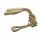The toy shop sold the same number of balls for 62 CZK and skipping ropes for 34 CZK. They income was 576 CZK. How many sold balls and how many skipping ropes?
• Average monthly salaryA total of 10 teachers work at one small school in Moravia. The monthly salary of each is 21,500 CZK or 21,800 CZK or 22,500 CZK according to their education and age. The average monthly salary for this school's teacher is 21 850 CZK. How many teachers of
• Three friendsThree friends divided the profit 104,650 CZK, so that for every 4 CZK, which got the first friend equals 5 crowns for second and for every 9 CZK, which got the second equals 16 CZK for third. Question: Who got the most and how much.
• BuingBrother got to buy 240 CZK and could buy for 1/8 what he wanted. Could he pay the rest of the purchase for 200 CZK?
• Scouts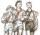Scouts purchased for camp cans two types total cost 1460 CZK. The first cans were for CZK 32 and the second for 25 CZK. How many cans purchased for each type?
• Coins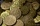The boy collected coins with value 5 CZK and 2 CZK when he had 50 pieces saved 190 CZK. How many he has each type of coin?
• 3 goods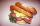Ms. Beránková bought in the shop 3 goods. Total paid for the purchase was 248 CZK. The first thing was 112 CZK and second 101 CZK. How much was the third thing?
• DiscountThe dress was discounted by CZK 115, which was 12% of the original price. What is the cost of the dress after the discount?
• Twelve ties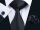The price of 12 ties before the discount was the same as 15 ties after discount. Calculate the price of 1 tie after discount, if you know that the discount price of one tie fell by 40 CZK?
• Chewing gums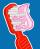For 3 chewing gums, you will pay 20 CZK less than 7 chewing gums. How much is 1 chewing gum and how much does a 5pcs package cost?
• ConcertOn a Concert were sold 150 tickets for CZK 360, 235 tickets for 240 CZK and 412 for 180 CZK. How much was the total revenues for tickets?
• Eva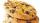When Eva buys 8 packages of cookies, 4 CZK left her. If she wanted to buy 10 packages, she would have to borrow 20 CZK. How much money has Eva in her wallet?